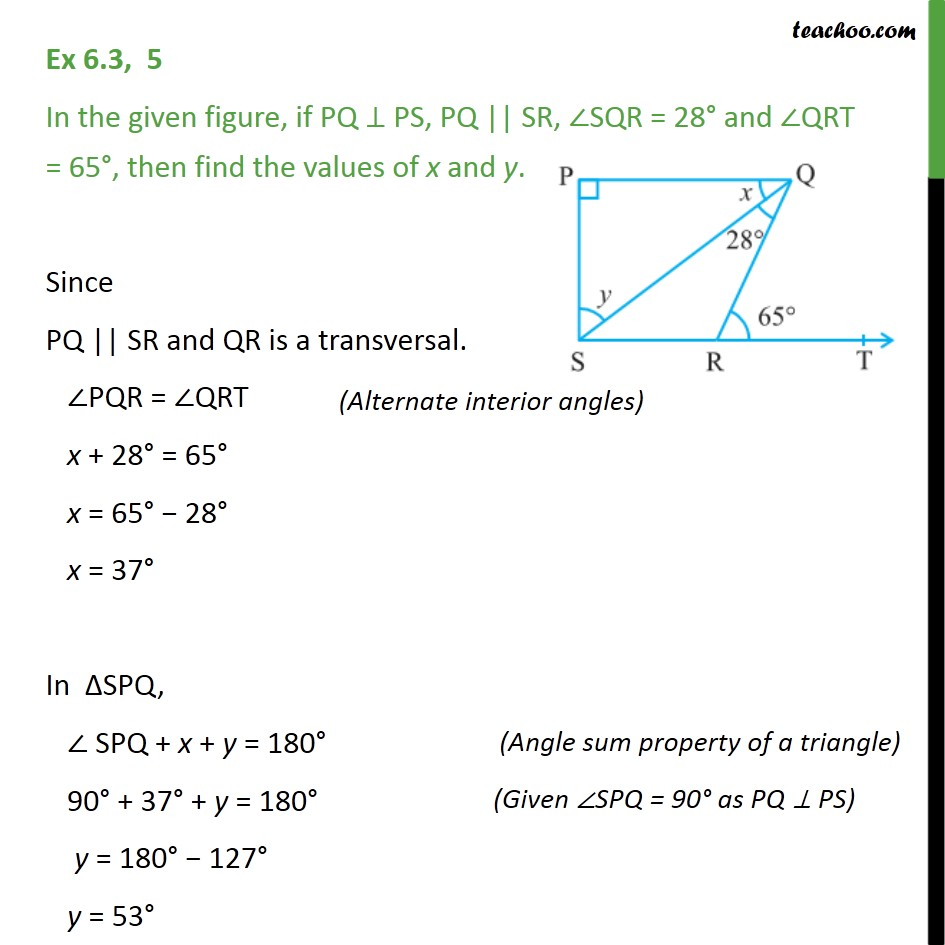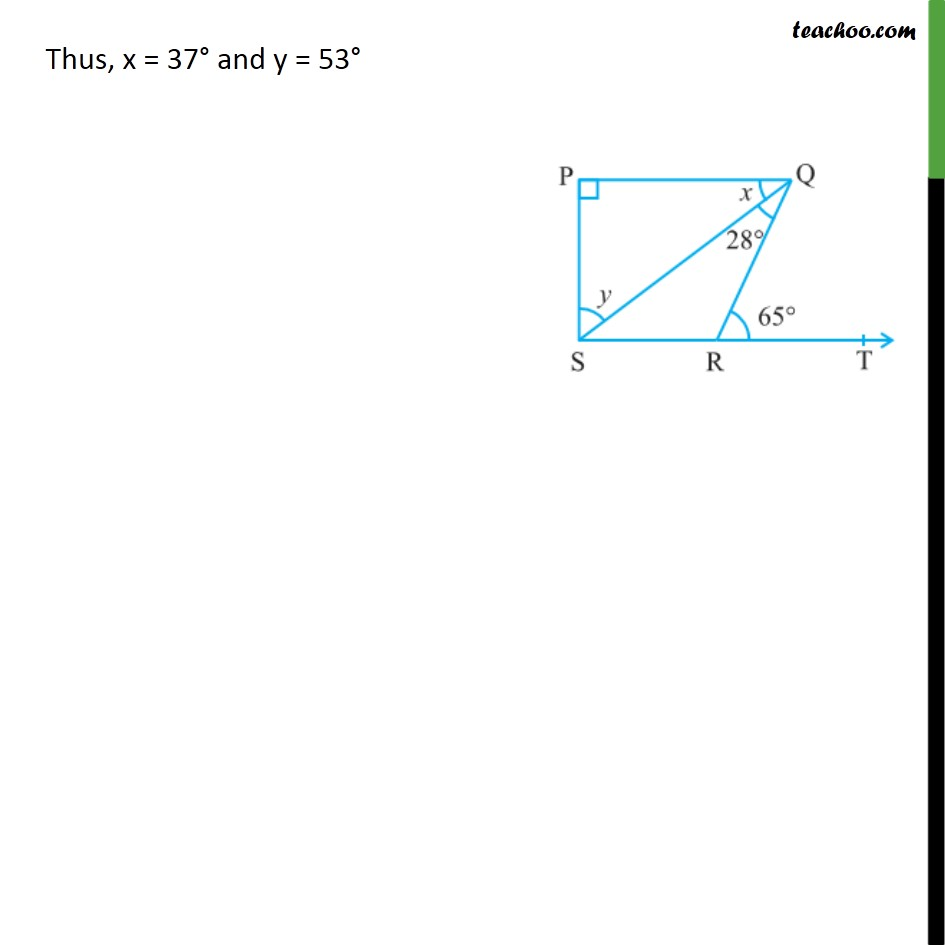Important Questions on Lines, Angles, Triangles

Chapter 6 Class 9 Lines and Angles
Serial order wiseLearn in your speed, with individual attention - Teachoo Maths 1-on-1 Class

### Transcript

Question 5 In the given figure, if PQ ⊥ PS, PQ || SR, ∠SQR = 28° and ∠QRT = 65°, then find the values of x and y. Since PQ || SR and QR is a transversal. ∠PQR = ∠QRT x + 28° = 65° x = 65° − 28° x = 37° In ΔSPQ, ∠ SPQ + x + y = 180° 90° + 37° + y = 180° y = 180° − 127° y = 53° Thus, x = 37° and y = 53°